## Example Questions

← Previous 1

### Example Question #1 : How To Find The Area Of A Square

How much more area does a square with a side of 2r have than a circle with a radius r?  Approximate  π by using 22/7.

4/7 square units

1/7 square units

6/7 square units

12/14 square units

6/7 square units

Explanation:

The area of a circle is given by A = πr2 or 22/7r2

The area of a square is given by A = s2 or (2r)2 = 4r2

Then subtract the area of the circle from the area of the square and get 6/7 square units.

### Example Question #1 : How To Find The Area Of A Square

If the perimeter of a square is 44 centimeters, what is the area of the square in square centimeters?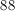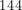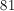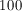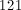Explanation:

Since the square's perimeter is 44, then each side is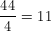.

Then in order to find the area, use the definition that the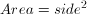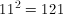### Example Question #1 : How To Find The Area Of A Square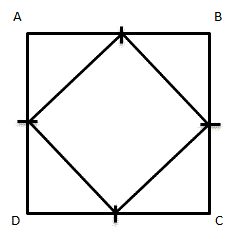Given square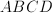, with midpoints on each side connected to form a new, smaller square.  How many times bigger is the area of the larger square than the smaller square?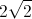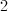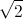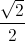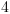Explanation:

Assume that the length of each midpoint is 1.  This means that the length of each side of the large square is 2, so the area of the larger square is 4 square units.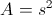To find the area of the smaller square, first find the length of each side.  Because the length of each midpoint is 1, each side of the smaller square is(use either the Pythagorean Theorem or notice that these right trianges are isoceles right trianges, so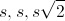can be used).

The area then of the smaller square is 2 square units.

Comparing the area of the two squares, the larger square is 2 times larger than the smaller square.

### Example Question #151 : Quadrilaterals

If a completely fenced-in square-shaped yard requires 140 feet of fence, what is the area, in square feet, of the lot?

4900

35

70

140

1225

1225

Explanation:

Since the yard is square in shape, we can divide the perimeter(140ft) by 4, giving us 35ft for each side. We then square 35 to give us the area, 1225 feet.

### Example Question #1 : How To Find The Area Of A Square

The perimeter of a square is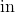. What is its area?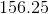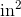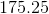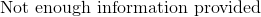Explanation:

The perimeter of a square is very easy to calculate. Since all of the sides are the same in length, it is merely: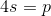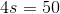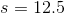From this, you can calculate the area merely by squaring the side's value: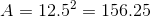### Example Question #11 : Squares

What is the area of a square with a side length of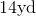?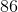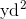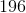Explanation:

The area of a square is very easy. You merely need to square the length of any given side. That is, the area is defined as: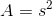For our data, this is: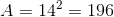### Example Question #391 : Geometry

What is the area of a square with a perimeter offt?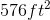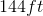Not enough information is given.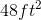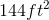Explanation:

For a square all the sides are equal, and there are four sides, so divide the perimeter by 4 to determine the side length.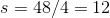.

Next to find the area of a square, square the side length: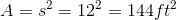.

### Example Question #151 : Quadrilaterals

Eric has 160 feet of fence for a parking lot he manages. If he is using all of the fencing, what is the area of the lot assuming it is square?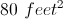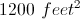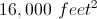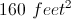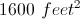Explanation:

The area of a square is equal to its length times its width, so we need to figure out how long each side of the parking lot is. Since a square has four sides we calculate each side by dividing its perimeter by four.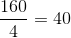Each side of the square lot will use 40 feet of fence.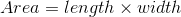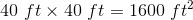.

### Example Question #1 : How To Find The Area Of A Square

A square garden is inscribed inside a circular cobblestone path. If the radius of the cobblestone path isfeet, what is the area of the garden?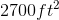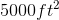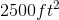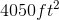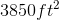Explanation:

If a square is inscribed inside a circle, the diameter of the circle is the diagonal of the square. Since we know the radius of the circle isfeet, the diameter must befeet. Thus, the diagonal of the square garden isfeet.

All squares have congruent sides; thus, the diagonal of a square creates two isosceles right triangles. The ratio of the lengths of the sides of an isosceles right triangle are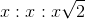, where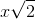is the hypotenuse. Thus, to find the length of a side of a square from the diagonal, we must divide by.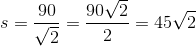The area of a square is, so if one side is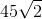, our area is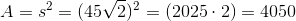Thus, the area of our square garden is### Example Question #1 : How To Find The Area Of A Square

Find the area of a square whose side length is.Explanation:

To find area, simply square the side length. Thus,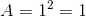← Previous 1

### All ACT Math Resources